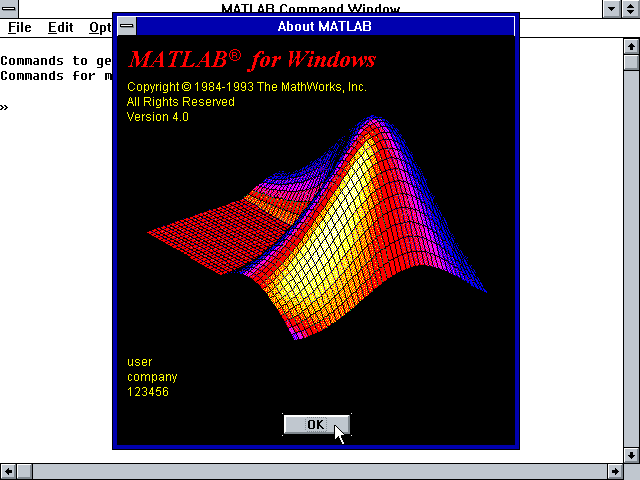# MatLab 4.x

edited December 2022MatLab 4.x

MatLab is a high speed, interactive programming utility for manipulating, calculating, and plotting complicated mathematical equations. It is considered easy to use for those familiar with typical algebra equations.